# 17

## Summary

### Factorization

The number 17 is a prime number.

### Properties and families

Property or family Parameter values First few numbers Proof of satisfaction/membership/containment
prime number it is the 7th prime number 2, 3, 5, 7, 11, 13, 17, 19, 23, 29, 31, 37, 41, 43, 47, 53, 59, 61, 67, 71, 73, 79, 83, 89, 97, [SHOW MORE]View list on OEIS A natural numberis prime if and only ifis not divisible by any prime less than or equal to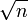. Since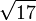is between 4 and 5, we only need to check divisibility by primes less than or equal to 4, i.e., we need to verify that 17 is not divisible by the primes 2 and 3.
Fermat number, Fermat prime, where, starts3, 5, 17, 257, 65537, 4294967297 View list on OEIS plug and check
Proth prime: prime of the formwith3, 5, 13, 17, 41, 97, 113, [SHOW MORE]View list on OEIS
regular prime sixth regular prime (2 is neither regular nor irregular) 3, 5, 7, 11, 13, 17, 19, 23, 29, 31, 41, 43, 47, 53, 61, [SHOW MORE]View list on OEIS
near-square prime of the form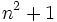(third prime of this form) 2, 5, 17, 37, 101, 197, 257, [SHOW MORE]View list on OEIS
lucky number of Euler fifth of six such numbers 2, 3, 5, 11, 17, 41 View list on OEIS A primeis a lucky number of Euler iff the ring of integers in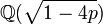is a unique factorization domain.

## Structure of integers mod 17

### Discrete logarithm

We can take 3 to be a primitive root mod 17, i.e., a generator for the multiplicative group of integers mod 17. With this, the discrete logarithm table from the multiplicative group mod 17 to the additive group mod 16 looks as follows:

Congruence class mod 17 (written as smallest positive integer) Congruence class mod 17 (written as smallest magnitude integer) Discrete logarithm to base 3, written as integer mod 16 Is it a primitive root mod 17 (if and only if the discrete log is relatively prime to 16)? Is it a quadratic residue or nonresidue mod 17 (residue if discrete log is even, nonresidue if odd)?
1 1 0 No quadratic residue
2 2 14 No quadratic residue
3 3 1 Yes quadratic nonresidue
4 4 12 No quadratic residue
5 5 5 Yes quadratic nonresidue
6 6 15 Yes quadratic nonresidue
7 7 11 Yes quadratic nonresidue
8 8 10 No quadratic residue
9 -8 2 No quadratic residue
10 -7 3 Yes quadratic nonresidue
11 -6 7 Yes quadratic nonresidue
12 -5 13 Yes quadratic nonresidue
13 -4 4 No quadratic residue
14 -3 9 Yes quadratic nonresidue
15 -2 6 No quadratic residue
16 -1 8 No quadratic residue
-- -- -- 8 Yes, 8 No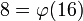,is Euler totient function
8 residues, 8 nonresidues. Equal numbers of both.

## Primitive root theory

### Primitive roots

The number of primitive roots equals the number of generators of the additive group of integers mod 16, which is the Euler totient function of 16, which is 8. Given any primitive root, the primitive roots are, i.e., the odd powers of. 17 is a Fermat prime so the primitive roots are precisely the quadratic nonresidues, see quadratic nonresidue equals primitive root for Fermat prime.

The explicit list of primitive roots is: 3,5,6,7,10,11,12,14.

We note the following:

### Significance of 10 being a primitive root

If 10 is a primitive root modulo a prime, then the primeis a full reptend prime in base 10, i.e., the decimal expansion ofhas a repeating block of the maximum possible length. The condition holds for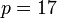, and the corresponding decimal expansion of 1/17 is: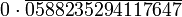The corresponding number: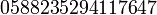has the property that it is a cyclic number, i.e., its first few multiples are obtained by cyclic permutations of its digits.

As noted above, the quadratic nonresidues coincide with the primitive roots and the quadratic residues are the remaining elements.

Further information: Formula for number of pairs of adjacent quadratic residues modulo a prime

For a primethat is congruent to 1 mod 4, the number of pairs of adjacent squares modis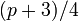and the number of adjacent (both nonzero) quadratic residues is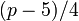. For, these numbers are respectively 5 and 3.

The 3 pairs of adjacent quadratic residues for 17 are: (1,2), (8,9), and (-2,-1) (which is the same as (15,16).

If we allow for 0 as one of the pair members, the 5 pairs of adjacent squares are: (0,1), (1,2), (8,9), and (-2,-1), and (-1,0).

### Paley graph and Ramsey theory

The structure of quadratic residues are nonresidues for the prime 17 has a combinatorial significance. Specifically, consider the Paley graph of integers mod 17, defined as the graph on the integers mod 17 where two vertices are connected if their difference is a quadratic residue mod 17. This is a self-complementary graph and it does not have a clique of size four, hence it can be used to show that the Ramsey numberis at least 18.

### Condition for 17 to be a quadratic residue modulo a prime

Whether or not 17 is a quadratic residue modulo a prime depends only on the congruence class of the prime modulo 68 (obtainedas 4 times 17). This follows from quadratic reciprocity for odd primes.

By Dirichlet's theorem on primes in arithmetic progressions, we thus find that there are infinitely many primesfor which 17 is a quadratic residue and infinitely many primesfor which 17 is a quadratic nonresidue.

### Square roots of -1

Since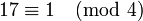, -1 has a square root mod 17. Its two square roots mod 17 are 4 and -4 (which is 13 mod 17).

We can also see this from the fact that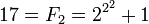, and that the square roots of -1 mod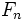are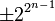.

## Waring representations

### Sums of squares

Item Value
unique (up to plus/minus and ordering) representation as sum of two squares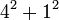. Note that existence and uniqueness both follow from it being a prime that is 1 mod 4.
This also corresponds to the factorization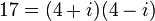in the ring of Gaussian integers.
representations as sum of three squares (up to ordering and plus/minus equivalence)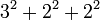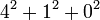### Sums of cubes

Item Value
representation as sum of two cubes not possible, because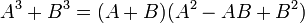is not possible
representation as sum of three cubes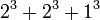is the only one using nonnegative inputs.
representation as sum of four cubes none using nonnegative inputs. Using both positive and negative inputs: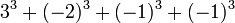### Sums of fourth powers

Item Value
representation as sum of two fourth powers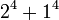## Cyclotomic theory

### Cyclotomic extension of primitive roots of unity

Fill this in later

### Constructibility of 17-gon

17 is a Fermat prime, hence the regular 17-gon is constructible by straightedge and compass. This is because the cyclotomic extension of adjoining 17th roots of unity can be obtained by taking successive quadratic extensions.

## Polynomials

### Prime-generating polynomials

Below are some polynomials that give prime numbers for small input values, which give the value 17 for suitable input choice.

Polynomial Degree Some values for which it generates primes Input valueat which it generates 17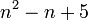2 all numbers 1,2,3,4, because 5 is one of the lucky numbers of Euler. 42 all numbers 1-10, because 11 is one of the lucky numbers of Euler. 32 all numbers 1-16, because 17 is one of the lucky numbers of Euler. 1

### Irreducible polynomials by Cohn's irreducibility criterion

By Cohn's irreducibility criterion, we know that if we write 17 in any base greater than or equal to 2, the corresponding polynomial is irreducible. We list here all the irreducible polynomials:

Base17 in baseCorresponding irreducible polynomial
2 10001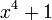3 122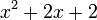4 1015 326 257 23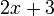8 21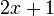9 1810 17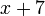11 16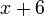12 15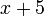13 1414 13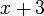15 1216 11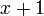## Multiples

### Interesting multiples

Number Prime factorization What's interesting about it
561 3 times 11 times 17 smallest Carmichael number, i.e., smallest absolute pseudoprime
1105 5 times 13 times 17 second Carmichael number, i.e., second absolute pseudoprime
2465 5 times 17 times 29 fourth Carmichael number, i.e., fourth absolute pseudoprime